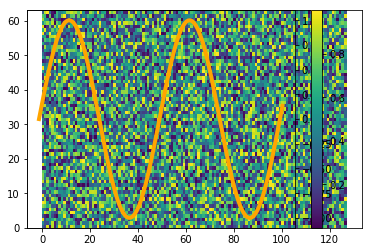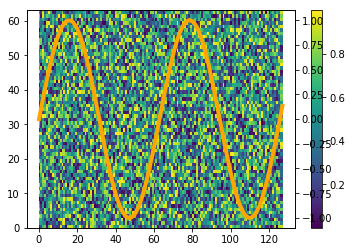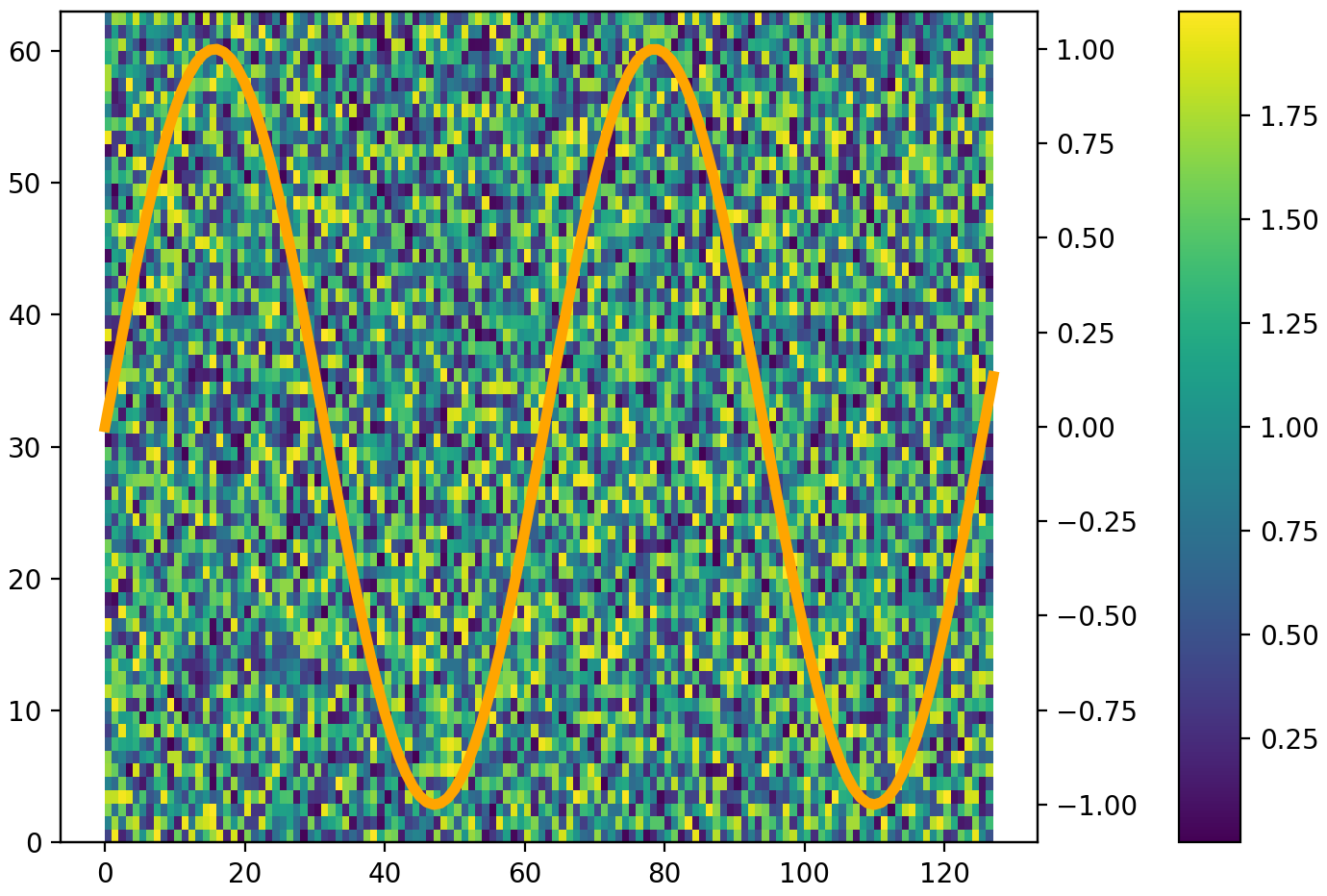# colorbar might shrink plots if used with twinx #8823

Closed
opened this issue Jun 29, 2017 · 5 comments

Projects
None yet

### Bug report

Changing plot alignment for different placing of colorbar() in twinx plot

If one combines a `pcolormesh` plot/`hist2d` plot with a standard `plot` by using `twinx`, the graph from the `plot` moves to wrong x values if the colorbar is called after the `plot` call. If it is called before, the alignment is fine.

I am not sure if this is a bug or just wrong usage.
However, one of the resulting graphs has a wrong extent that might lead to misinterpretations.

Code for reproduction

```import numpy as np
import matplotlib.pyplot as plt

data = np.random.random((64, 128)) * 2.0
x = np.arange(128)
y = np.arange(64)
plt.pcolormesh(x, y, data)

#plt.colorbar() --> here it works fine
plt.twinx()
plt.colorbar()
# --> here it gives wrong x values for the orange plot

plt.plot(x, np.sin(x/10), color="orange", lw=4)

plt.show()```

Actual outcomeNext to the confusing layout the plot shows actually a wrong graph:
the orange plot just goes to around x=100 but should go to x=128.
(The axis of the colorbar is correct and shows the range of `data`.)

Expected outcomeIf `colorbar` is called before `twinx`, the orange graph has the correct extent.

I am not sure if this is a bug or a wrong usage of matplotlib. In any case the wrong extent of the (in this case) orange plot is a dangerous behavior that might lead to misinterpretations of the data.

Matplotlib version

• Operating System: Ubuntu 14.04.1
• Matplotlib Version: 2.0.0rc1
• Python Version: 3.4.3
• Jupyter Version: 1.0.0 (I am using `%matplotlib inline`)
• Other Libraries: numpy 1.12.0b1

I installed matplotlib via pip.

Member

### efiring commented Jun 29, 2017

 I would call it a shortcoming of the twinx implementation: it makes a second Axes at the same position as the first, but it has no mechanism for ensuring that if the position of either is subsequently changed, the position of the other is also changed to match it. Ideally, the position of both Axes would be the same object so that it would not be necessary to use callbacks to keep them synchronized. There are several attributes related to position, so I'm not sure whether this would be a simple change, a difficult one, or a complete can of worms.

Contributor

### jklymak commented Dec 11, 2017

 #9082 addresses this (though you can't use the pyplot interface) ```data = np.random.random((64, 128)) * 2.0 x = np.arange(128) y = np.arange(64) fig, ax = plt.subplots(constrained_layout=True) pcm = ax.pcolormesh(x, y, data) #plt.colorbar() --> here it works fine ax2 = ax.twinx() fig.colorbar(pcm) # --> here it gives wrong x values for the orange plot ax2.plot(x, np.sin(x/10), color="orange", lw=4) plt.show()```Author

### PrometheusPi commented Dec 12, 2017

 @jklymak Thanks for posting this. I will cherry pick your pull request.
Member

### efiring commented Jan 21, 2018

 This is also addressed by #10033.
Member

### efiring commented Jan 26, 2018

 Closed by #10033.